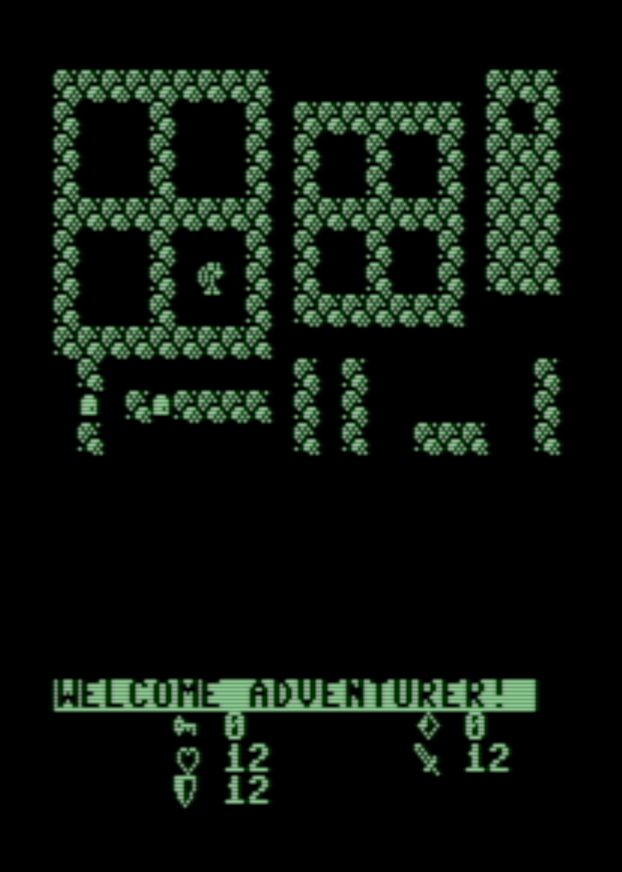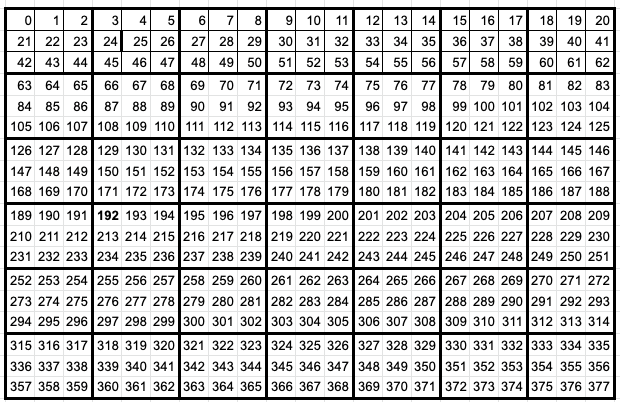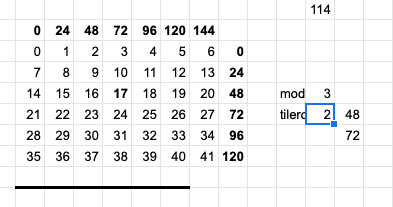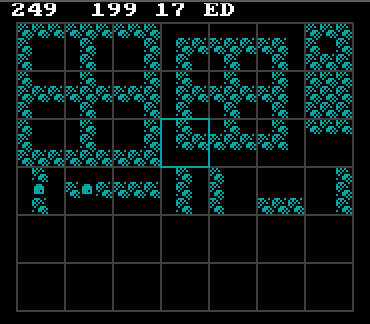# Character Cell to Tiles and Back Again Algorithm

in #programminglast yearThankfully, I managed to get the C64 side (and PET and Vic 20!) loading the tiles and maps without having to change too much but it did come down to a lot of scribbled notes and head scratching.

Math, basically.

I hate math, especially when it comes to doing math that a 1mhz machine can do within 1/24 of a second.

Therefore I am writing this partly for my own use later down the line when I can't for the life of me remember what the heck I was doing and how I had figured it out.

The situation at hand is my tiles are made up of a serialized set of characters that will eventually be implemented as 3x3 tiles.

So, for example, character 192 is the top left corner of tile number 22.

While this makes loading and saving the binary file trivially easy, it makes my head spin working out how to go from that list of characters to convert into those 3x3 tiles.

I have certainly gotten spoiled over the years by dictionaries, custom types, and multi-dimensional arrays of any size I want.## Character Cell to Tile

To get from tile number to character set, we need to identify the first character of the tile.

We do this by first getting the row.

``````=mod(tile,7)
=((O26-O27)/7)
=O28*(63)
=Q28+(O27*3)
``````

This gives us the remainder of the tile divided by the number of tiles on a row, which is the X position.

We then take the remainder away from the tile number, and divide that by 7, giving us the tile row.

Multiplying the row by 64 (which is 3 * 7 * 3) gives us the start of the character row we need, and then we add back the X position to get the starting character.

Whew!

## Going the Other Way

In my map editor, I need to click on an X and Y pixel coordinate provided by the mouse and then extrapolate that into a tile number from 0 to 41.

The tiles start at pixel (10, 10), so the provided X and Y need to remove those extra 10 pixels of padding before we can calculate anything accurately.

There are 24 pixels height in each tile (3x8), so we divide the Y by that number to get our tile row.

We also need to divide the horizontal coordinate by the same amount (the tiles are 24x24 square), then add the X result to the Y multiplied by the number of tiles on a row, which is 7.

``````
tmp_row = _MOUSEY - 10
tmp_row = INT(tmp_row / 24)

selected_tile = INT((_MOUSEX - 10) / 24) + (tmp_row * 7)
``````

## Bringing it Together

All that remains is to draw a box around the selected tile, which is of course back to pixel coordinates.

I get the impression I am doing this the hard way, but on we go!

``````                remainder = selected_tile MOD 7
tilerow = (selected_tile - remainder) / 7
y = tilerow * 24
x = remainder * 24
CALL draw_grid
LINE (x + 10, y + 10)-(x + 34, y + 34), blue, B
``````

We need, again, to know which column and row we are looking at in terms of 3x3 tiles laid out in a 7x6 grid, so we take the selected_tile and find the remainder from dividing by 7 (the number of tiles across).Next we get the row by taking the remainder away and dividing by 7.

Now we have the column and row, we need to multiply by 24 (3x8 pixels remember), and draw a pretty blue box around the selected item!Sort:
last year Reveal Comment